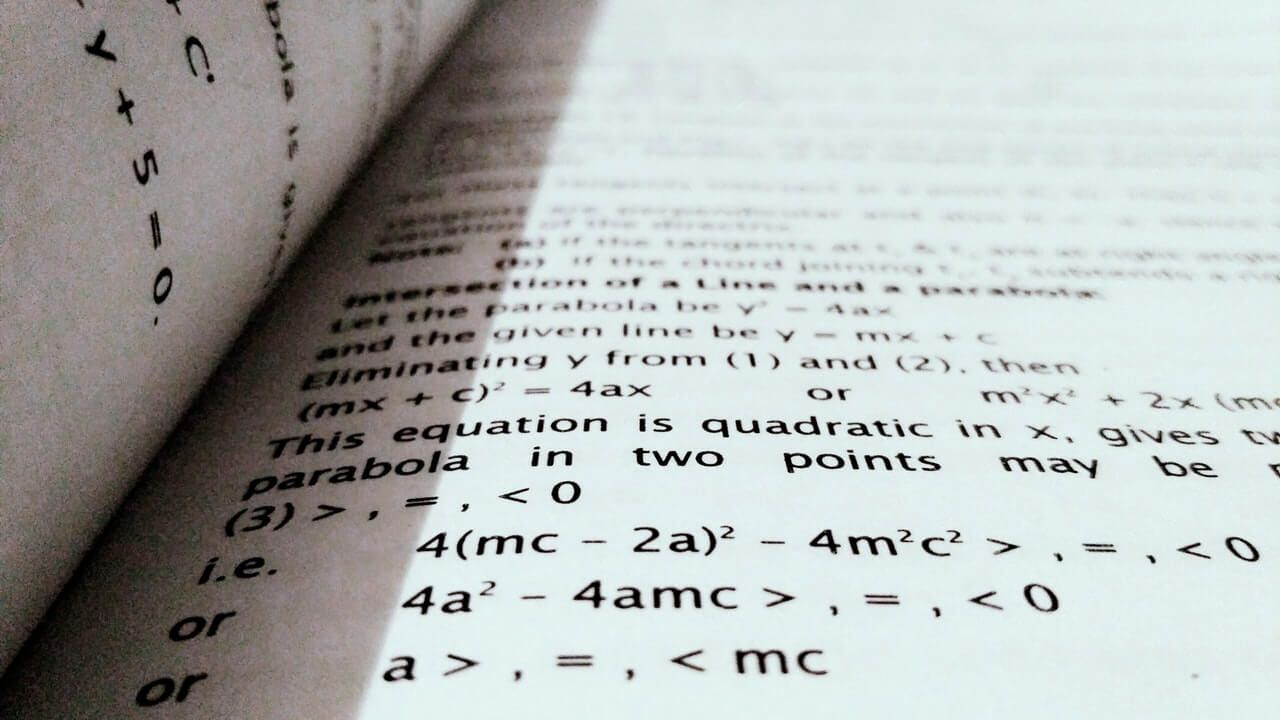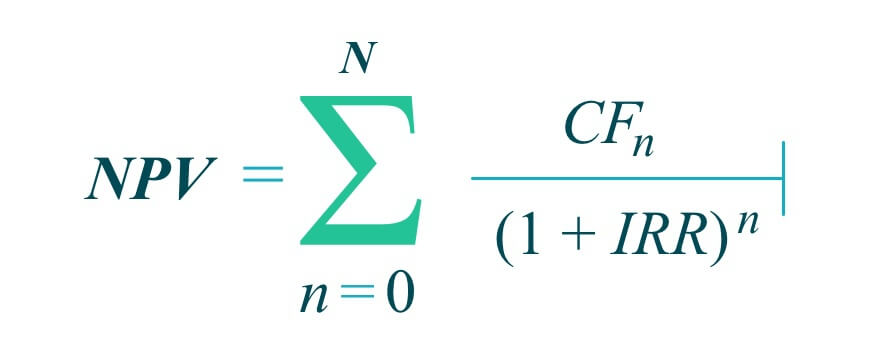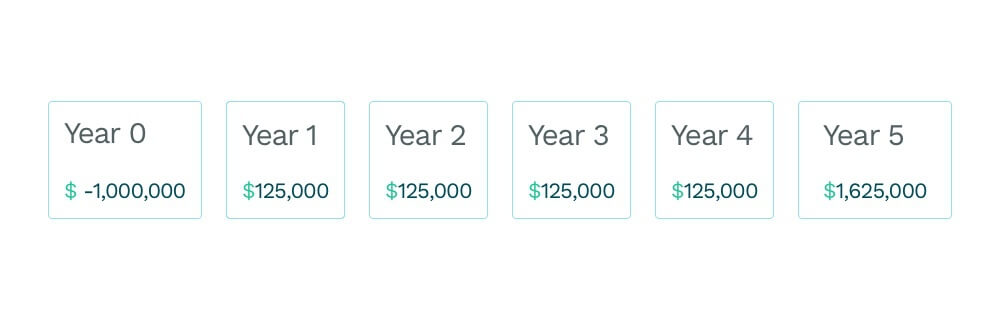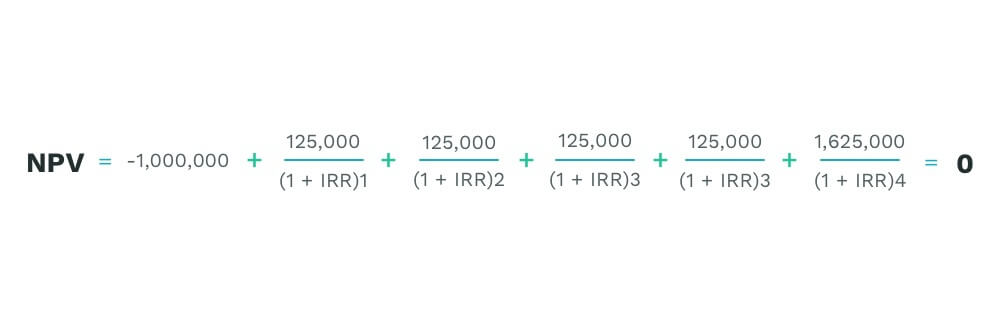# What is the IRR formula and why it matters for real estate investmentsby Meredith Galante, posted in Investment Strategy

When investing in real estate, you’ll want to know if you’re going to make a profit from your holdings.

Unfortunately, it’s not as simple as it sounds to figure out. One of the best ways to understand if you will get a good return on your property is to use the Internal Rate of Return (IRR) formula, which is used across several industries including real estate.

## What is the IRR formula?

The IRR formula helps investors understand their yearly earnings. Its result is based on a percentage of the investor’s original investment and what they hope to one day sell the home for. Essentially, it’s a formula that helps investors realize what they’ll earn overtime on their investment. This result can be used to compare to see if they will earn more in a particular real estate investment versus putting their money in a more traditional investment or a different property.

Looking at the IRR formula might give you flashbacks to the anxiety you had during high school calculus, but after understanding the formula a bit further, you’ll be able to calculate the IRR yourself.To help you make sense of the formula, here’s what all those letters stand for:

• N = The number of years you own the property
• CFn = Your current cash flow from the property
• n = The current year/stage you’re in while calculating the formula
• NPV = Net Present Value
• IRR = Internal rate of return

## What do I need to calculate my IRR?In lamens terms, to calculate your IRR correctly you need to input real data into the formula.

First, you’ll need to evaluate if you plan to put any additional investment into the property, such as re-doing the kitchen or upgrading appliances, etc. If so, this will negatively affect your cash flow in year 1.

Next, you’ll need to consider your cash flow from any rental income. This is the monthly rent your tenant will pay you to live there.

Then, you’ll want to think about how long you plan to own the investment property. After all, this formula is about money valued over time. How long you own the property will affect how many years you’ll receive rental income and could affect the potential sale price.

## How do I calculate the IRR formula?

After you’ve gathered all the input data needed, it’s time to find out what your expected annual return will be. While using the formula, all money will be calculated in the present-day value of cash.

Let’s say you buy a property for \$1 million. Yearly, you’ll earn \$125,000 from rental income for four years. Then, you plan to sell the property in five years for \$1.5 million. Your cash flow would look like this:With these assumptions, set the NPV to zero to determine the IRR. You’ll see that year four has increased cash flow because the profit from the sale is added to the rental income.

The IRR formula would look like this:The result is an internal rate of return of 21.61 percent.

If the math still seems too complicated, you can use Microsoft Excel, as it has a few built-in formulas to help investors calculate the IRR themselves.

## What are the drawbacks of using the IRR formula?

While the IRR formula gives investors a prediction of what the return can be on a property, it can be difficult to calculate it properly without the right tools and because there is a lot of guesswork involved.

The IRR formula is most useful when trying to compare investments over the same length of time. So, it’s conversely least useful when you’re trying to compare investments that would last a different length of time.

To calculate your IRR, you also need to do some guessing about your sale price and how long you do plan to own the property. For example, if the market tanks, you might be off about your sale price and your calculated IRR won’t be accurate.

The IRR also doesn’t account for any costly repairs investors might incur, such as a new roof that could cost upwards of \$10,000 or a tenant leaving unexpectedly which would mean no rental income that month.

## Why do investors need to care about the IRR formula?

Smart investors want to earn money on anything they’re investing in. Utilizing the IRR formula helps them consider if the property will generate the returns they hoped for compared to a different investment.

However, all the assumptions made about the data inputted into the formula must be as close to accurate as possible, otherwise, the results will be skewed.

The IRR is not the only tool an investor would use to evaluate a real estate property’s up or downside, but it helps provide a window into predicted returns and the value of money over time and uses a discounted cash flow analysis. Investors can use other metrics such as cash flow, cash on return, or cap rates.

The IRR also shows investors when they’d receive the money and how much of it. So for example, a shorter-term investment with a higher rate of return may seem better, a longer-term real estate investment could have a bigger final cumulative payout. Every investors’ goals are different, but the comparison will help them make better investment decisions.